# Axiomatic Analysis of Elementary Indices

Economics, Economic / Financial Data

Before reading this feature, it may help if you first read: Elementary Indices.

Elementary index calculation methods can be tested against various axioms, to investigate whether or not they have certain desirable properties.

Some axioms are more important than others.

## Axioms

For each of the following axioms:
n is the sample size of the good being surveyed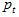are the prices of the good at the current period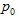are the prices of the good at the base period
P is the elementary price index and is a function of the current and base period prices

### Continuity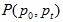is a continuous function of n positive base period prices and n positive current period prices.

### Identity

The index at the base period equals one.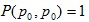### Monotonicity in Current Period Pricesif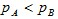### Monotonicity in Base Period Prices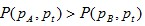if### Proportionality in Current Period Prices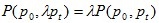if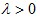### Inverse Proportionality in Base Period Prices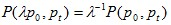if### Mean Value Test

The price index lies between the smallest and biggest price ratios.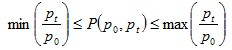### Symmetric Treatment of Establishment / Products

If there is a change in the ordering of establishments (or products within an establishment) the price quotations are obtained from for the two periods, the elementary index remains unchanged.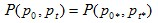where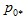and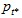denote the same permutation of the components ofand.

### Price-Bouncing Test

A more generalised (and more controversial) version of the previous axiom, the price-bouncing test allows for different permutations between the base and current periods.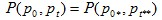whereanddenote possibly different permutations of the components ofand.

### Time Reversal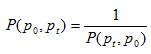### Circularity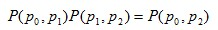### Commensurability

The units of measurement for each product have no effect on the price index.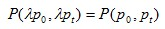## How the elementary indices compare

 Dutot Jevons Carli Harmonic Mean of Price Relatives Carruthers / Sellwood / Ward / Dalén Ratio of Harmonic Means Continuity Y Y Y Y Y Y Identity Y Y Y Y Y Y Monotonicity in Current Period Prices Y Y Y Y Y Y Monotonicity in Base Period Prices Y Y Y Y Y Y Proportionality in Current Period Prices Y Y Y Y Y Y Inverse Proportionality in Base Period Prices Y Y Y Y Y Y Mean Value Test Y Y Y Y Y Y Symmetric Treatment of Establishment / Products Y Y Y Y Y Y Price-Bouncing Test Y Y N N N Y Time Reversal Y Y N N Y Y Circularity Y Y N N N Y Commensurability N Y Y Y Y N

Jevons index satisfies all of the axioms described here.  In some countries (including USA and New Zealand), Jevons index has been used more in recent years, replacing the Dutot index at least for some categories.

A problem with the Dutot index (and ratio of harmonic means) is that it doesn’t satisfy the commensurability axiom.  This means that if the units of measurement change for a product, it would affect the price index.  For some products, this won’t be a problem.

The Carli index and the harmonic mean of price relatives don’t satisfy the price bouncing test, time reversal or circularity.  The inability for time reversal is the biggest of these problems, but can be solved by taking the geometric mean of the two indices i.e. by using a Carruthers / Sellwood / Ward / Dalén index.  The inability for time reversal has meant the Carli index is upwardly biased, and the harmonic mean of price relatives is downwardly biased.